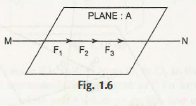Coplanar force means the foces on the same plane and collinear force means the forces on the same line. Thus, at the moment means when the different forces act on the same line in a plane, then it is called coplanar collinear forces.

It means if the three different forces F1, F2 and F3 lie in the same line and in the same plane, then it will be coplanar and collinear at a time.The forces can be determined through graphical and analytical forces. So the proper representation through graphical force is important and more than that graph line must intersect the points in such a way that all related forces make a single line. In case the points do not intersect properly and make a straight line, then it will give you a wrong outcome and it will not be considered as a collinear in a coplanar. So, it is very important to have in the same line and in the same plane.

Links of Next Mechanical Engineering Topics:-### Customer Reviews

My Homework Help
Rated 5.0 out of 5 based on 510 customer reviews at